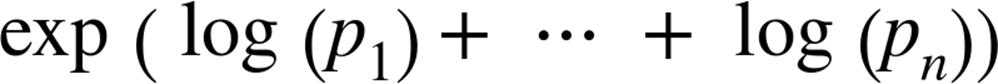﻿ ﻿Naive Bayes - Data Science from Scratch: First Principles with Python (2015)

# Data Science from Scratch: First Principles with Python (2015)

### Chapter 13.Naive Bayes

It is well for the heart to be naive and for the mind not to be.

Anatole France

# A Really Dumb Spam FilterA More Sophisticated Spam FilterImplementation
``def` `tokenize(message):``
`    `message` `=` `message.lower()`                       `# convert to lowercase``
`    `all_words` `=` `re.findall("[a-z0-9']+",` `message)`   `# extract the words``
`    `return` `set(all_words)`                           `# remove duplicates``
``def` `count_words(training_set):``
`    `"""training set consists of pairs (message, is_spam)"""``
`    `counts` `=` `defaultdict(lambda:` `[``0,` `0])``
`    `for` `message,` `is_spam` `in` `training_set:``
`        `for` `word` `in` `tokenize(message):``
`            `counts[word][0` `if` `is_spam` `else` `1]` `+=` `1``
`    `return` `counts``
``def` `word_probabilities(counts,` `total_spams,` `total_non_spams,` `k=0.5):``
`    `"""turn the word_counts into a list of triplets``
``    w, p(w | spam) and p(w | ~spam)"""``
`    `return` `[(``w,``
`             `(``spam` `+` `k)` `/` `(``total_spams` `+` `2` `*` `k),``
`             `(``non_spam` `+` `k)` `/` `(``total_non_spams` `+` `2` `*` `k))``
`             `for` `w,` `(``spam,` `non_spam)` `in` `counts.iteritems()]``
``def` `spam_probability(word_probs,` `message):``
`    `message_words` `=` `tokenize(message)``
`    `log_prob_if_spam` `=` `log_prob_if_not_spam` `=` `0.0``
` `
`    `# iterate through each word in our vocabulary``
`    `for` `word,` `prob_if_spam,` `prob_if_not_spam` `in` `word_probs:``
` `
`        `# if *word* appears in the message,``
`        `# add the log probability of seeing it``
`        `if` `word` `in` `message_words:``
`            `log_prob_if_spam` `+=` `math.log(prob_if_spam)``
`            `log_prob_if_not_spam` `+=` `math.log(prob_if_not_spam)``
` `
`        `# if *word* doesn't appear in the message``
`        `# add the log probability of _not_ seeing it``
`        `# which is log(1 - probability of seeing it)``
`        `else:``
`            `log_prob_if_spam` `+=` `math.log(1.0` `-` `prob_if_spam)``
`            `log_prob_if_not_spam` `+=` `math.log(1.0` `-` `prob_if_not_spam)``
` `
`    `prob_if_spam` `=` `math.exp(log_prob_if_spam)``
`    `prob_if_not_spam` `=` `math.exp(log_prob_if_not_spam)``
`    `return` `prob_if_spam` `/` `(``prob_if_spam` `+` `prob_if_not_spam)``
``class` `NaiveBayesClassifier:``
` `
`    `def` `__init__(self,` `k=0.5):``
`        `self.k` `=` `k``
`        `self.word_probs` `=` `[]``
` `
`    `def` `train(self,` `training_set):``
` `
`        `# count spam and non-spam messages``
`        `num_spams` `=` `len([is_spam``
`                         `for` `message,` `is_spam` `in` `training_set``
`                         `if` `is_spam])``
`        `num_non_spams` `=` `len(training_set)` `-` `num_spams``
` `
`        `# run training data through our "pipeline"``
`        `word_counts` `=` `count_words(training_set)``
`        `self.word_probs` `=` `word_probabilities(word_counts,``
`                                             `num_spams,``
`                                             `num_non_spams,``
`                                             `self.k)``
` `
`    `def` `classify(self,` `message):``
`        `return` `spam_probability(self.word_probs,` `message)``

# Testing Our Model

``import` `glob,` `re``
` `
``# modify the path with wherever you've put the files``
``path` `=` `r"C:\spam\*\*"``
` `
``data` `=` `[]``
` `
``# glob.glob returns every filename that matches the wildcarded path``
``for` `fn` `in` `glob.glob(path):``
`    `is_spam` `=` `"ham"` `not` `in` `fn``
` `
`    `with` `open(fn,'r')` `as` `file:``
`        `for` `line` `in` `file:``
`            `if` `line.startswith("Subject:"):``
`                `# remove the leading "Subject: " and keep what's left``
`                `subject` `=` `re.sub(r"^Subject: ",` `"",` `line).strip()``
`                `data.append((subject,` `is_spam))``
``random.seed(0)`      `# just so you get the same answers as me``
``train_data,` `test_data` `=` `split_data(data,` `0.75)``
` `
``classifier` `=` `NaiveBayesClassifier()``
``classifier.train(train_data)``
``# triplets (subject, actual is_spam, predicted spam probability)``
``classified` `=` `[(``subject,` `is_spam,` `classifier.classify(subject))``
`              `for` `subject,` `is_spam` `in` `test_data]``
` `
``# assume that spam_probability > 0.5 corresponds to spam prediction``
``# and count the combinations of (actual is_spam, predicted is_spam)``
``counts` `=` `Counter((is_spam,` `spam_probability` `>` `0.5)``
`                 `for` `_,` `is_spam,` `spam_probability` `in` `classified)``
``# sort by spam_probability from smallest to largest``
``classified.sort(key=lambda` `row:` `row)``
` `
``# the highest predicted spam probabilities among the non-spams``
``spammiest_hams` `=` `filter(lambda` `row:` `not` `row,` `classified)[-5:]``
` `
``# the lowest predicted spam probabilities among the actual spams``
``hammiest_spams` `=` `filter(lambda` `row:` `row,` `classified)[:5]``
``def` `p_spam_given_word(word_prob):``
`    `"""uses bayes's theorem to compute p(spam | message contains word)"""``
` `
`    `# word_prob is one of the triplets produced by word_probabilities``
`    `word,` `prob_if_spam,` `prob_if_not_spam` `=` `word_prob``
`    `return` `prob_if_spam` `/` `(``prob_if_spam` `+` `prob_if_not_spam)``
` `
``words` `=` `sorted(classifier.word_probs,` `key=p_spam_given_word)``
` `
``spammiest_words` `=` `words[-5:]``
``hammiest_words` `=` `words[:5]``

§ Look at the message content, not just the subject line. You’ll have to be careful how you deal with the message headers.

§ Our classifier takes into account every word that appears in the training set, even words that appear only once. Modify the classifier to accept an optional `min_count` threshhold and ignore tokens that don’t appear at least that many times.

§ The tokenizer has no notion of similar words (e.g., “cheap” and “cheapest”). Modify the classifier to take an optional `stemmer` function that converts words to equivalence classes of words. For example, a really simple stemmer function might be:

`§  `def` `drop_final_s(word):``
`    `return` `re.sub("s\$",` `"",` `word)``

Creating a good stemmer function is hard. People frequently use the Porter Stemmer.

§ Although our features are all of the form “message contains word,” there’s no reason why this has to be the case. In our implementation, we could add extra features like “message contains a number” by creating phony tokens like contains:number and modifying the `tokenizer` to emit them when appropriate.

# For Further Exploration

§ Paul Graham’s articles “A Plan for Spam” and “Better Bayesian Filtering” (are interesting and) give more insight into the ideas behind building spam filters.

§ `scikit-learn` contains a `BernoulliNB` model that implements the same Naive Bayes algorithm we implemented here, as well as other variations on the model.

﻿